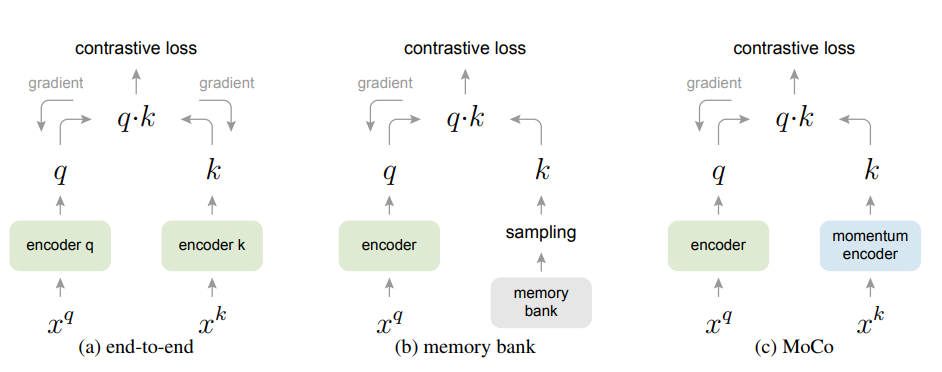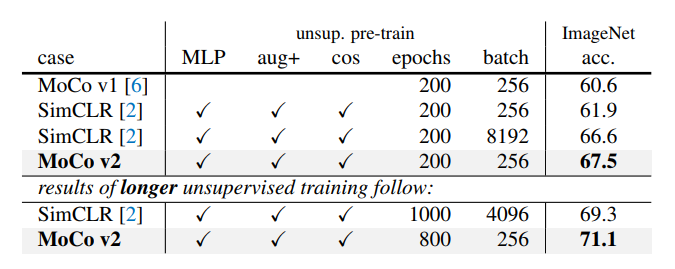@Team 2020-09-20T15:26:27.000000Z 字数 5250 阅读 2445

# CV中的无监督学习方法：MoCo

我是小将

## 对比学习## Moco

Moco的核心就是维护一个动态的queue作为字典，然后通过momentum update方式更新字典keys编码网络，其PyTorch伪代码如下所示：

# f_q, f_k: encoder networks for query and key# queue: dictionary as a queue of K keys (CxK)# m: momentum# t: temperaturef_k.params = f_q.params # initializefor x in loader: # load a minibatch x with N samples    x_q = aug(x) # a randomly augmented version    x_k = aug(x) # another randomly augmented version    q = f_q.forward(x_q) # queries: NxC    k = f_k.forward(x_k) # keys: NxC    k = k.detach() # no gradient to keys    # positive logits: Nx1    l_pos = bmm(q.view(N,1,C), k.view(N,C,1))    # negative logits: NxK    l_neg = mm(q.view(N,C), queue.view(C,K))    # logits: Nx(1+K)    logits = cat([l_pos, l_neg], dim=1)    # contrastive loss, Eqn.(1)    labels = zeros(N) # positives are the 0-th, so GT label is 0    loss = CrossEntropyLoss(logits/t, labels)    # SGD update: query network    loss.backward()    update(f_q.params)    # momentum update: key network    f_k.params = m*f_k.params+(1-m)*f_q.params    # update dictionary    enqueue(queue, k) # enqueue the current minibatch    dequeue(queue) # dequeue the earliest minibatch# bmm: batch matrix multiplication; mm: matrix multiplication; cat: concatenation

augmentation = [    transforms.RandomResizedCrop(224, scale=(0.2, 1.)),    transforms.RandomGrayscale(p=0.2),    transforms.ColorJitter(0.4, 0.4, 0.4, 0.4),    transforms.RandomHorizontalFlip(),    transforms.ToTensor(),    normalize]

# compute query features，query计算过程不做改动q = self.encoder_q(im_q)  # queries: NxCq = nn.functional.normalize(q, dim=1)# key的计算要先shuffle 样本，这样同一张上query和key的BN层采用不同的样本计算得到，避免信息泄露with torch.no_grads():    # shuffle for making use of BN    im_k, idx_unshuffle = self._batch_shuffle_ddp(im_k)    k = self.encoder_k(im_k)  # keys: NxC    k = nn.functional.normalize(k, dim=1)    # undo shuffle    k = self._batch_unshuffle_ddp(k, idx_unshuffle)

augmentation = [            transforms.RandomResizedCrop(224, scale=(0.2, 1.)),            transforms.RandomApply([                transforms.ColorJitter(0.4, 0.4, 0.4, 0.1)  # not strengthened            ], p=0.8),            transforms.RandomGrayscale(p=0.2),            transforms.RandomApply([moco.loader.GaussianBlur([.1, 2.])], p=0.5),            transforms.RandomHorizontalFlip(),            transforms.ToTensor(),            normalize        ]## 参考• 私有
• 公开
• 删除